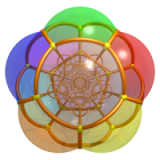# 称硬币问题

${\bf s} = H{\bf x}^T$，则 ${\bf s}$ 是一个 $r\times 1$ 的向量，${\bf s}$ 叫做 ${\bf x}$ 的校验子 (syndrome)。这里的神奇之处在于我们可以从天平的状态推出 ${\bf s}$ 的值，然后反解出 ${\bf x}$

1. 如果天平是平衡的，由于两边硬币数目相同，那么两边一定都是真币，假币不出现，即 $y_i=0$，从而 $s_1=0$
2. 如果天平左边向上翘起，由于两边硬币数目相同，这有两种可能：假币要轻一些，且放在了左边；或者假币重一些，放在了右边。即 $x_i=y_i=1$ 或者 $x_i=y_i=2$，总之 $s_1=x_iy_i=1$ （注意在 $\mathbb{F}_3$$2\times2=1$）。
3. 如果天平右边向上翘起，由于两边硬币数目相同，这有两种可能：假币要轻一些，且放在了右边；或者假币重一些，放在了左边。即 $x_i=1$$y_i=2$ 或者 $x_i=2$$y_i=1$，总之 $s_1=x_iy_i=2$

${\bf s}$ 与错误位置对应向量是共线的，所以只要找到 $H$ 的列向量中与 ${\bf s}$ 共线的那一个 ${\bf c}_i$，则 $i$ 就是出错的硬币，根据 ${\bf s}={\bf c}_i$ 还是 ${\bf s}=2{\bf c}_i$ 可以得出这枚硬币是轻了还是重了。但是这样做有一个前提，就是 $H$ 的列向量必须两两不共线，这才能唯一地确定 $i$于是我们的问题转化为设计一个 $r\times 12$ 的矩阵 $H$$H$ 的每一行中 1 和 2 的个数相同，且 $H$ 的任何两列互不共线，这个 $H$ 叫做校验矩阵

1 2 3 4 5 6 7 8 9 10 11 12
0 0 0 0 1 1 1 1 2 2 2 2
0 1 1 1 0 0 0 1 2 2 1 1
1 0 1 2 0 1 2 0 2 1 0 2

1 2 3 4 5 6 7 8 9 10 11 12
0 0 0 0 1 1 1 1 2 2 2 2
0 1 2 2 0 0 0 1 2 2 1 1
1 0 2 1 0 1 2 0 2 1 0 2

 第一次 5, 6, 7, 8 9, 10, 11, 12 第二次 2, 8, 11, 12 3, 4, 9, 10 第三次 1, 4, 6, 10 3, 7, 9, 12

1. 要能够从天平的状态得出有用的信息，必须在天平两边放置同样数目的硬币，即 $H$ 的每一行有相同个数的 1 和 2，这时我们可以推断出校验子 ${\bf s}$ 的值；

2. 另一方面 ${\bf s}$ 必然与 $H$ 的某个列向量共线，所以只要 $H$ 的列向量互不共线，就可以唯一地确定错误位置对应的列向量 ${\bf c}_i$ 并得出错误值。

# 小白鼠找毒药问题

${\bf x}=(x_1,x_2,\ldots, x_{100})$，其中 $x_i\in\mathbb{F}_2$$x_i=1$ 当且仅当第 $i$ 瓶药水是毒药。根据已知 ${\bf x}$ 中恰好有一个位置为 1，即发生了一个错误，我们只要检测出这个错误位置即可。

$\mathbb{F}_2$ 上总共有 $2^r$ 个不同的向量，于是 $2^r\geq 100$$r\geq 7$，从而 7 只小白鼠就足够了。只要从 $\mathbb{F}_2^7$ 中任选 100 个向量，将它们作为 $H$ 的列向量即可。根据校验子 ${\bf s}$ 找到 $H$ 与之相同的列向量 ${\bf c}_i={\bf s}$，则第 $i$ 瓶药水就是毒药。

# Leetcode 上的一道题目

There are 1000 buckets, one and only one of them contains poison, the rest are filled with water. They all look the same. If a pig drinks that poison it will die within 15 minutes. What is the minimum amount of pigs you need to figure out which bucket contains the poison within one hour?

${\bf x}=(x_1,x_2,\ldots, x_{1000})$，其中 $x_i\in\mathbb{F}_5$，每个 $x_i$ 只有 0, 1 两种取值，且 $x_i=1$ 当且仅当第 $i$ 桶水有毒。根据已知 ${\bf x}$ 中恰好有一个位置为 1，即发生了一个错误，由于错误的值是已知的，我们仍然只要检测这个错误位置即可。

|